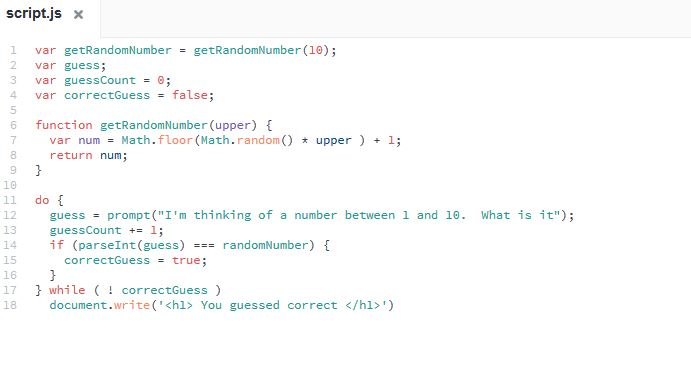## Welcome to the Treehouse Community

Want to collaborate on code errors? Have bugs you need feedback on? Looking for an extra set of eyes on your latest project? Get support with fellow developers, designers, and programmers of all backgrounds and skill levels here with the Treehouse Community!

### Looking to learn something new?

Treehouse offers a seven day free trial for new students. Get access to thousands of hours of content and join thousands of Treehouse students and alumni in the community today.# My prompt will not loop

Can't figure it out for the life of meSTAFF

Well I see a problem on your first line which affects the rest of your code. You have this:

```var getRandomNumber = getRandomNumber(10);
```

But later you have this:

```if(parseInt(guess === randomNumber) {
```

I sincerely believe that your first line should be this:

```var randomNumber = getRandomNumber(10);
```

Try this change and see if you can get some different results!Thanks Jennifer. I'll take this is as a hint that I need to take more breaks.Hi,

Here's my code for this exercise since I didn't notice the issue in yours. Hope it will help.

Does the code at least run once ?

```var randomNumber = getRandomNumber(10);
var guess;
var guessCount = 0;
var correctGuess = false;

function getRandomNumber( upper ) {
var num = Math.floor(Math.random() * upper) + 1;
return num;
}

do {
guess = prompt("I am thinking of a number between 1 and 10. What is it?");
guessCount += 1;
if (parseInt(guess) === randomNumber) {
correctGuess = true;
}
} while ( ! correctGuess )
document.write("<h1>You guessed the number !</h1>");
document.write("It took you " + guessCount + " tries to guess the number " + randomNumber);
```